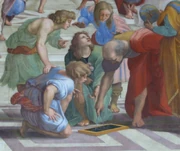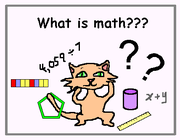## FANDOM

902 Pages

Definition:

1. "The Queen of Sciences", Gauss.
2.Euclid, 3 century B.C., as depicted by Raphael in "The School of Athens" "The study of number and shape", the era of Greek mathematics from 500 B.C. to 300 A.D.
3. In the mid-1600's, with the invention of calculus by Newton and Leibniz, mathematics became "the study of number, shape, motion, change, and space."
4. Galileo Galilei (1564–1642) said, "The universe cannot be read until we have learned the language and become familiar with the characters in which it is written. It is written in mathematical language, and the letters are triangles, circles and other geometrical figures, without which means it is humanly impossible to comprehend a single word. Without these, one is wandering about in a dark labyrinth." Thus, math is the universal "language" in which the Universe is "written".
5. "By the end of the nineteenth century, mathematics had become the study of number, shape, motion, change, and space, and of the mathematical tools that are used in this study."
6. Devlin describes mathematics as the "science of patterns... patterns of counting, patterns of reasoning and communicating, patterns of motion and change, patterns of shape, patterns of symmetry and regularity, and patterns of position."
7. Bertrand Russell has quipped, "Mathematics may be defined as the subject in which we never know what we are talking about, nor whether what we are saying is true."
8. From an elementary school volunteer: "Math is more than a subject we learn in school. Math is every breath we take and every second of the day. From the moment we wake up in the morning, math is the core of everything we do..."
9.From a 13-year-old: "Math is the entire world simplified on a piece of paper... Math is ingeniousness morphed into a tiny simple formula so we can harness its fantastic powers."
10. From a 12-year-old: "Math is the universal language of the world."
11. Wikipedia: "Since the pioneering work of Giuseppe Peano (1858–1932), David Hilbert (1862–1943), and others on axiomatic systems in the late 19th century, it has become customary to view mathematical research as establishing truth by rigorous deduction from appropriately chosen axioms and definitions."
12. French mathematician Claire Voisin states "There is creative drive in mathematics, it's all about movement trying to express itself."

So, we can say that the answer to "what is math" develops together with our general picture of the world. This is true of every science, for example logic. It is never "THE logic", but develops together with research methods used by people seeking knowledge. Mathematics is akin to logic. It is methodology of science, or of knowing in general. Methodology develops together with different methods used in various ways of knowing.

History of mathematics:

1. Sketch on history of mathematics 2013

Directions in math:

Mathematical logic

Fractals

Chaos theory

Number theory

Topology

Lie groups

Differential equations

Names in math:

Newton, 1643-1727

Leibniz, 1646-1716

Boole

Cantor

Frege

Tarsky

Morgan

David Hilbert, 1862-1943

Norbert Weiner, 1894-1964

John von Newmann, 1903-1957

Kurt Godel, 1906-1978

Benois Mandelbrodt, 1924-2010

Gregory Perel'man (Rus.), b. 1968

Present day math:

Other languages:

Математика

All items (10)

Community content is available under CC-BY-SA unless otherwise noted.